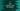# C program to find the square and cube of a number# Find square and cube of a number in C :

Let me show you how to write a C program to find the square and cube of a number. The program will take the number as input from the user, find out the square, cube, and print out the result to the user. We can use functions or we can directly calculate and print the values.

## Example 1: Find the square and cube of a number without using a function :

``````#include <stdio.h>

int main()
{
int no;
printf("Enter a number : ");
scanf("%d", &no);

printf("Square of %d is %d\n", no, (no * no));
printf("Cube of %d is %d\n", no, (no * no * no));
}``````

Sample output :

``````Enter a number : 3
Square of 3 is 9
Cube of 3 is 27

Enter a number : 2
Square of 2 is 4
Cube of 2 is 8``````

## Example 2: Find the square and cube using a function :

``````#include <stdio.h>

int findSquare(int n)
{
return n * n;
}

int findCube(int n)
{
return n * n * n;
}

int main()
{
int no;
printf("Enter a number : ");
scanf("%d", &no);

printf("Square of %d is %d\n", no, findSquare(no));
printf("Cube of %d is %d\n", no, findCube(no));
}``````

In this example, we have created two functions to find out the square and cube of a number. These functions take one integer and return the square and cube for that number.

The main benefit of using a function is that you can call it from anywhere in the program. It will be one single place with your logical things. If you change the function, this will reflect in all the places you are using it.